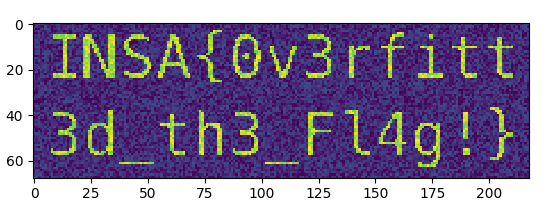5 May 2019

# INS'hAck 2019 - Neurovision

We found this strange file from an AI Startup. Maybe it contains sensitive information…

We get a keras model (.h5) for a neural network with just 1 output (sigmoid activation). The input layer seems to be some kind of image, matrix of 68x218.

``````Input layer :  Tensor("flatten_1_input:0", shape=(?, 68, 218), dtype=float32)
Output layer: Tensor("dense_1/Sigmoid:0", shape=(?, 1), dtype=float32)
``````

The objective is to generate a random image and alter it so that the output of the network goes in the direction we want.

We calculate the gradient for the first layer, insted of the intermediate layers. This way we know how to evolve the image so that the output converges on our desired value.

First, load the model an extract the input and output layers:

``````model = keras.models.load_model('neurovision-2d327377b559adb7fc04e0c3ee5c950c')
model_input_layer = model.layers.input
model_output_layer = model.layers[-1].output
``````

Define the cost and gradient functions:

``````# Our cost function is the mean squared error between the actual output and 1, our target value.
cost_fn = keras.losses.mean_squared_error(model_output_layer, 1)

# Function that returns the cost and gradient, when given an input
``````

Then the iterations to evolve the initially random image:

``````target_cost = 0.1
learning_rate = 20
# Generate a list of 1 random image of 218x68 pixels.
hacked_image=np.random.rand(1,68,218)

while True:

if cost < target_cost:
break

# Update the image
``````plt.imshow(hacked_image)